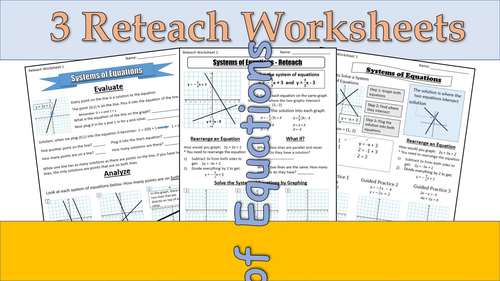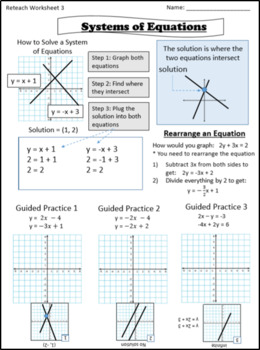# Solve Systems of Equations by Graphing - Reteach WorksheetsSubject
Resource Type
File Type

PDF

(440 KB)
Product Rating
4.0
(1 Rating)
Standards
• Product Description
• StandardsNEW

Each worksheet reinforces the skill through a discovery of the rules. Students are guided through practice problems that help them analyze the concepts and draw inferences on them.

Each worksheet also has practice problems with immediate feedback, followed by some independent practice problems for assessment.

Solve a simple system consisting of a linear equation and a quadratic equation in two variables algebraically and graphically. For example, find the points of intersection between the line 𝘺 = –3𝘹 and the circle 𝘹² + 𝘺² = 3.
Solve systems of linear equations exactly and approximately (e.g., with graphs), focusing on pairs of linear equations in two variables.
Solve real-world and mathematical problems leading to two linear equations in two variables. For example, given coordinates for two pairs of points, determine whether the line through the first pair of points intersects the line through the second pair.
Solve systems of two linear equations in two variables algebraically, and estimate solutions by graphing the equations. Solve simple cases by inspection. For example, 3𝘹 + 2𝘺 = 5 and 3𝘹 + 2𝘺 = 6 have no solution because 3𝘹 + 2𝘺 cannot simultaneously be 5 and 6.
Understand that solutions to a system of two linear equations in two variables correspond to points of intersection of their graphs, because points of intersection satisfy both equations simultaneously.
Total Pages
N/A
N/A
Teaching Duration
N/A
Report this Resource to TpT
Reported resources will be reviewed by our team. Report this resource to let us know if this resource violates TpT’s content guidelines.